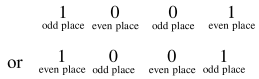# Let a computer program generate

Question:

Let a computer program generate only the digits 0 and 1 to form a string of binary numbers with probability of occurrence of 0 at even places be $\frac{1}{2}$ and probability of occurrence of 0 at the odd place be $\frac{1}{3}$. Then the probability that ' 10 ' is followed by ' 01 ' is equal to :

1. $\frac{1}{18}$

2. $\frac{1}{3}$

3. $\frac{1}{6}$

4. $\frac{1}{9}$

Correct Option: , 4

Solution:$\Rightarrow\left(\frac{1}{2} \cdot \frac{1}{3} \cdot \frac{1}{2} \cdot \frac{2}{3}\right)+\left(\frac{2}{2} \cdot \frac{1}{2} \cdot \frac{1}{3} \cdot \frac{1}{2}\right)$

$\Rightarrow \frac{1}{9}$